Technical Article

# AC-DC Converters - Disassembling a Linear Power Supply

December 22, 2015 by David Williams

## An AC-DC wall adaptor gets disassembled to see what components are used to build it, then the purpose of each component is analyzed.

### Introduction

Wall adapter AC-DC converters (rectifiers) are such common devices that no one pays much attention to them unless they go missing and you can’t get the DC power that you need. On the surface, these rectifiers are fairly simple devices and I am sure that you have some level of understanding of how they work.  Bear with me as I lay out the basic operation of AC-DC converters in this article. In subsequent articles, I will describe some of the more complicated topics related to AC-DC converters such as power factor, harmonic distortion, and reliability.

The AC-DC converter in the image below expects an input of 120Vac at 60 Hz and provides an output of about 12VDC (it is an unregulated converter and so the output will vary with the load) and up to 1 Amp of current. The maximum input power is 20W.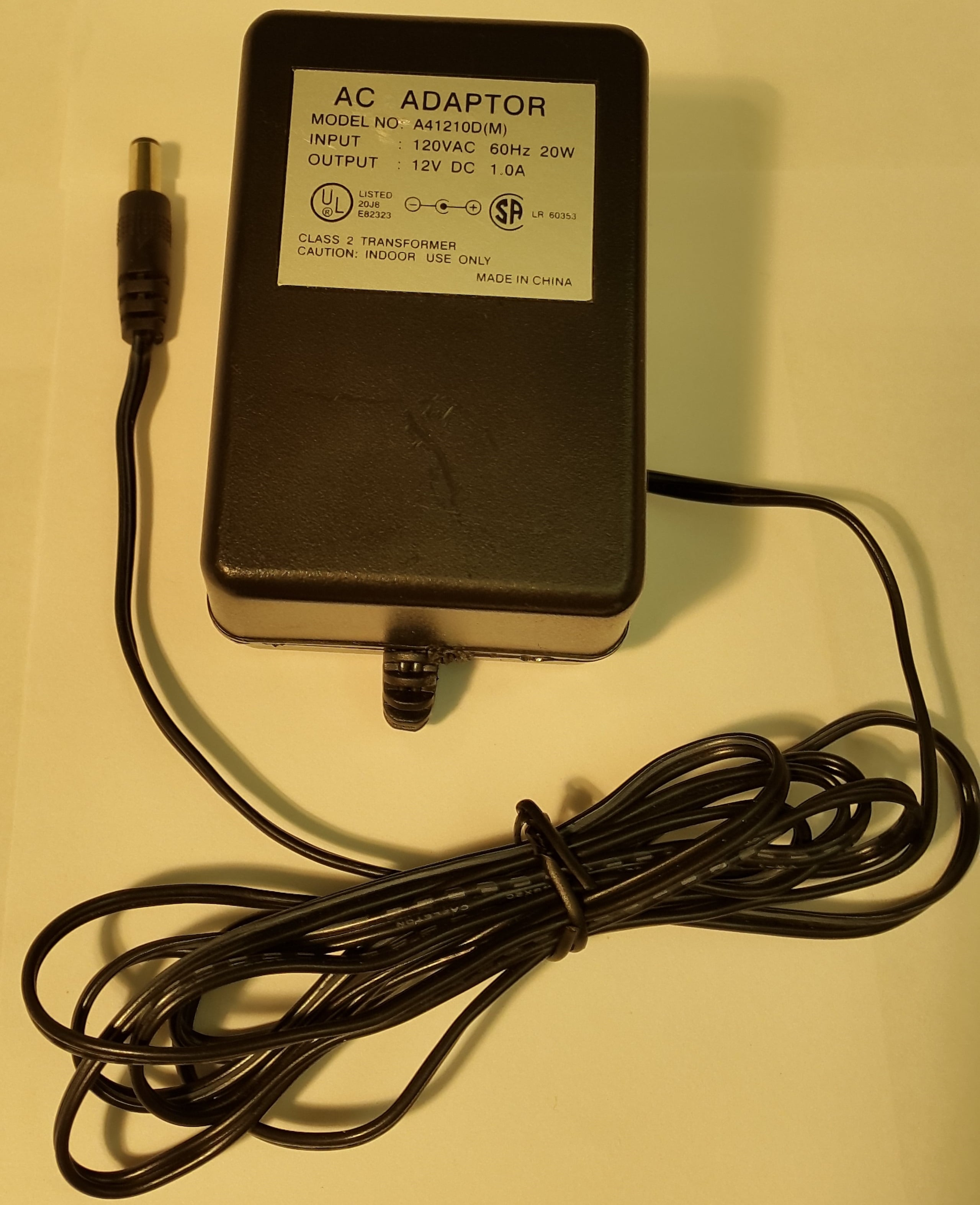Figure 1. A 20W, 120VAC to 12VDC Adaptor

Let’s assume we know nothing about the way they work and only have the capability of measuring voltages into and out of the device. Measuring the input voltage and the output voltage would give the following results: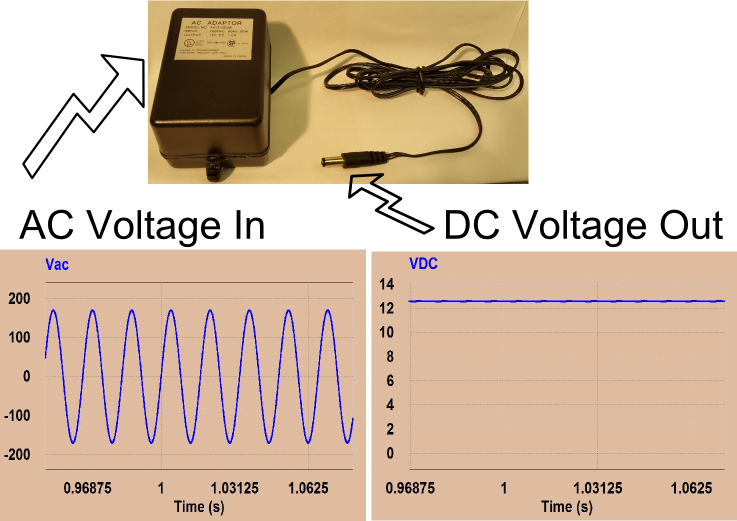Figure 2. Input and Output Voltages of AC-DC Adaptor

If we were just users of the wall adapter, that would be just about all that we need to know. The only other important thing would be to check the polarity of the plug and the socket to make sure they match (in this case, the center of the plug is positive). We are not just users of electronics though; we are curious electrical engineers, so let’s open up this converter to get a better idea of what is inside.

As you can see in figures 3 and 4, there is not much to this 120VAC to 12VDC adaptor.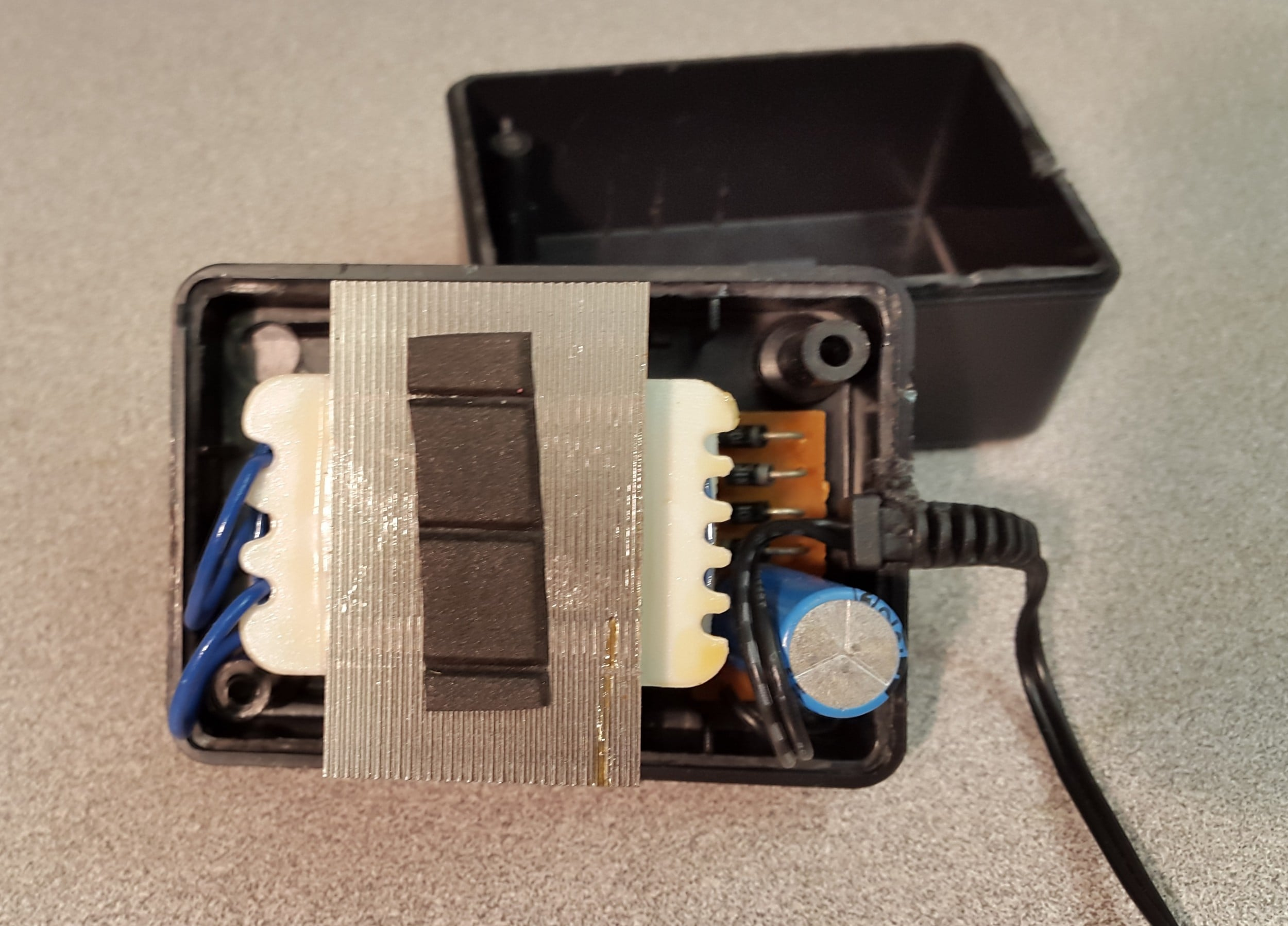Figure 3. Top View of the Inside of the AC Adaptor

#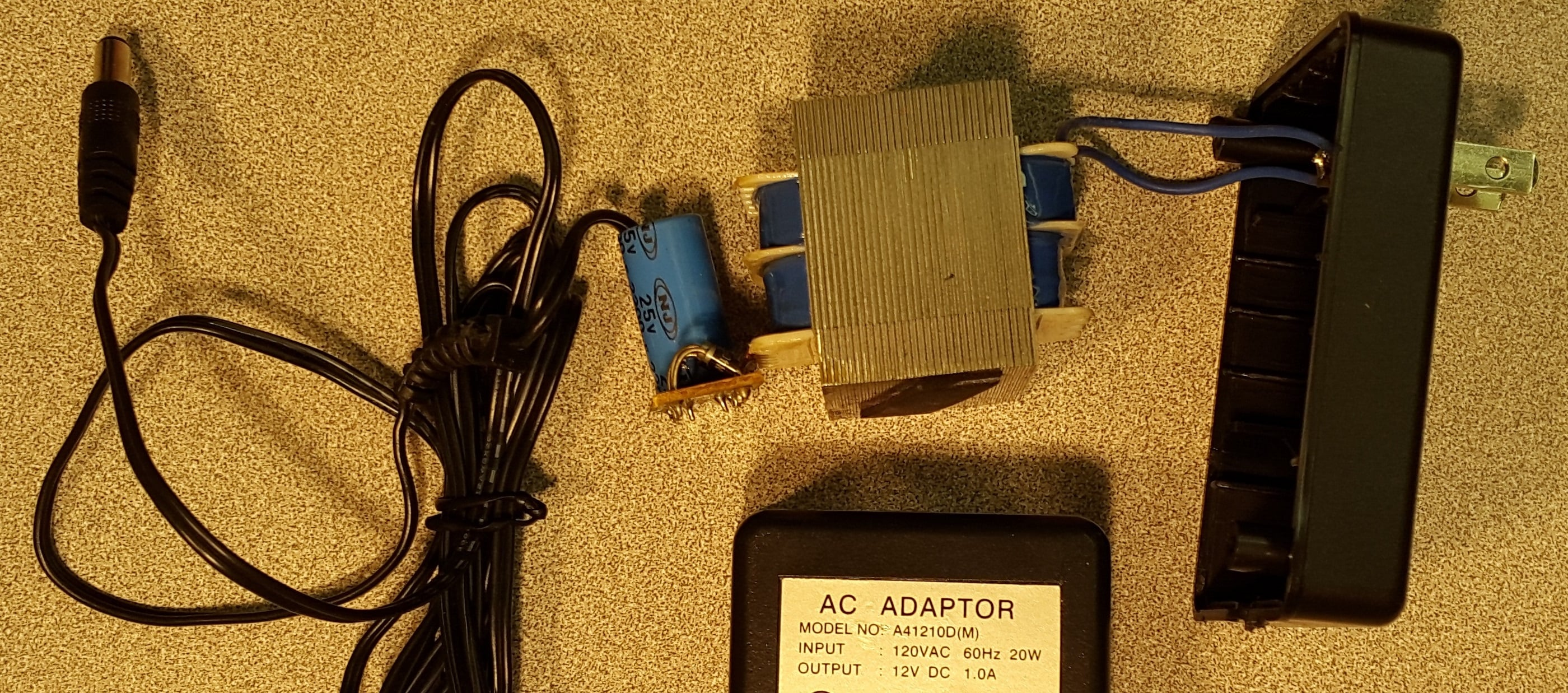Looking at figure 4 and going from right (input) to left (output), there is a two-prong plug, connected to some wires going to the primary coils of a transformer. Then connected to the secondary of the transformer is a full bridge diode rectifier, followed by a filtering capacitor, and finally a center positive output jack. In the sections that follow the purpose of each of these components will be analyzed to show how the AC input voltage ultimately gets converted into a 12V DC voltage.

### Transformer

Figure 5 shows the same adaptor seen from the side. The blue wires on the right are the inputs from the two-prong wall connection and they connect directly to the primary of the transformer. The output from the secondary can be seen at the lower left of the transformer as two small copper wires. The purpose of the transformer is to step the AC voltage down from the 120VRMS from the wall outlet to a voltage that is closer to the required DC voltage.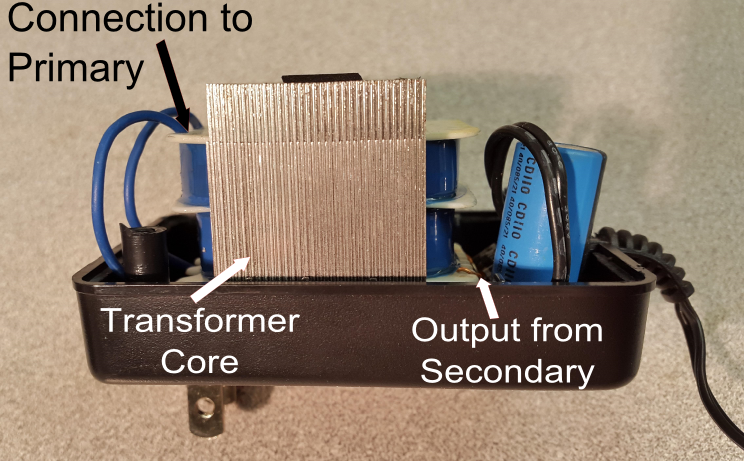Figure 5. Side View of AC Adaptor with Transformer Labeled

If you ignore all of the non-ideal properties of transformers, they are very simple devices. The general idea is that there are two (usually large) coils of wire that are electrically isolated, but magnetically coupled together. The input side of the transformer is called the primary and the output side is called the secondary. Alternating current passes through the primary coil which creates an alternating magnetic flux in the transformer core. This alternating magnetic flux in turn induces a voltage in the coils of the secondary. The ratio of the number of loops in the primary coil to the number of loops in the secondary coil is equal to the ratio of the input AC voltage to the output AC voltage. In equation form this relationship is:

$\frac{N_{P}}{N_{S}} = \frac{V_{P}}{V_{S}}$

$N_{P}$ is the number of loops in the primary coil, $V_{P}$ is the voltage applied to the primary coil, $N_{S}$ is the number of loops in the secondary coil and $V_{S}$ is the voltage out of the secondary coil.

Figure 6 shows a cartoon representation of the coupling of the primary winding to the secondary winding through the transformer core. The geometry of the core of the AC adaptor from Figure 5 is different from this diagram's geometry, but the principle is the same.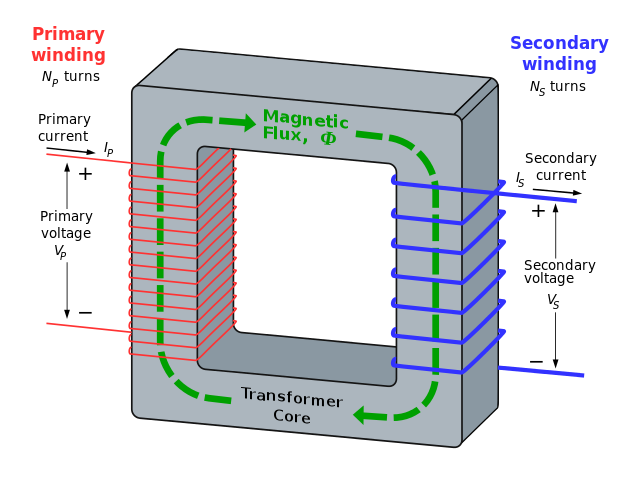Figure 6. Transformer Diagram

Transformers do not generate energy or (ideally) use any energy; they are entirely passive devices that simply step-up or step-down the AC voltage. Since they are not generating or producing power, the power in to the transformer must equal the power out. What that means is that if your transformer steps up the voltage, then it must step down the current to maintain the relationship $Power_{in} = Power_{out}$ (or $V_{in} \times I_{in} = V_{out} \times I_{out}$). Using the fact that power into the primary (P) equals the power out of the secondary (S), we can derive an equation relating current in the primary to current in the secondary:

$Power_{(P)} = Power_{(S)}$     which means

$V_{P} \times I_{P} = V_{S} \times I_{S}$

substituting in $V_{S} = \frac{N_{S}}{N_{P}} \times V_{P}$  gives

$V_{P} \times I_{P} = \frac{N_{S}}{N_{P}} \times V_{P} \times I_{S}$

the $V_{P}$ terms cancel out and the primary to secondary current relationship becomes:

$\frac{I_{P}}{I_{S}} = \frac{N_{S}}{N_{P}}$

The relationship between the primary and secondary voltage of this particular adaptor can be seen in Figure 7.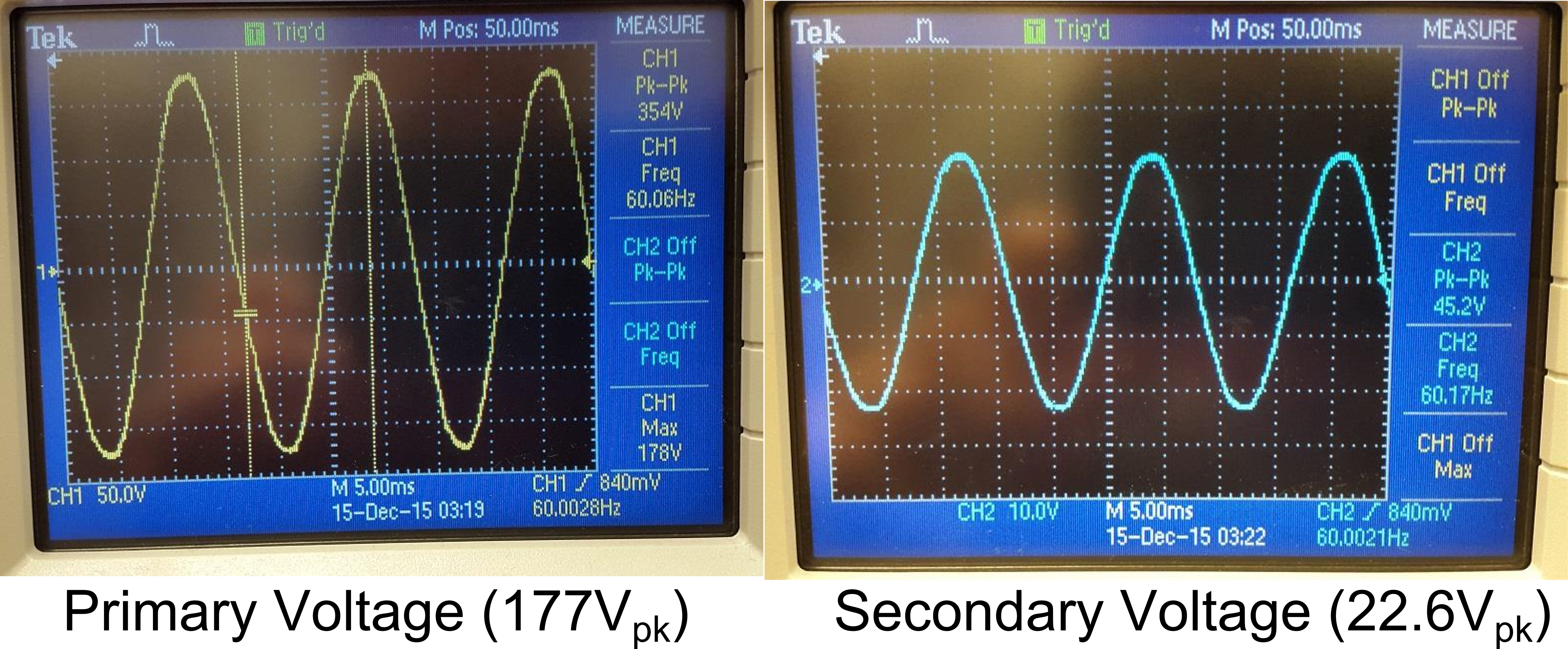Figure 7. The Primary and Secondary Voltages of the Transformer Shown on an Oscilloscope.

This figure shows that the primary voltage is 354Vp-p (177Vp or 125VRMS) and the output is 45.2Vp-p (22.6Vp or 16VRMS). The voltage ratio is about 7.8 (354/45.6) which means that the ratio of the number of turns in the primary to the secondary is also 7.8.

There are a few non-ideal characteristics of transformers such as wire resistance, core saturation, magnetizing inductance and hysteresis that must be considered when more precise modeling of the system must be done.  For the purposes of this article, we will just focus on the fact that a 177Vp AC voltage comes into the transformer and a 22.6Vp AC voltage goes out.

### The Bridge Rectifier

The next stage in the wall adapter is the bridge rectifier. This device takes the AC output of the transformer and converts it into a DC voltage. It does this using an arrangement of diodes that force the current to pass through the load in one direction only. Figure 8 shows the diodes in the adaptor along with a schematic representation of how the diodes are connected together.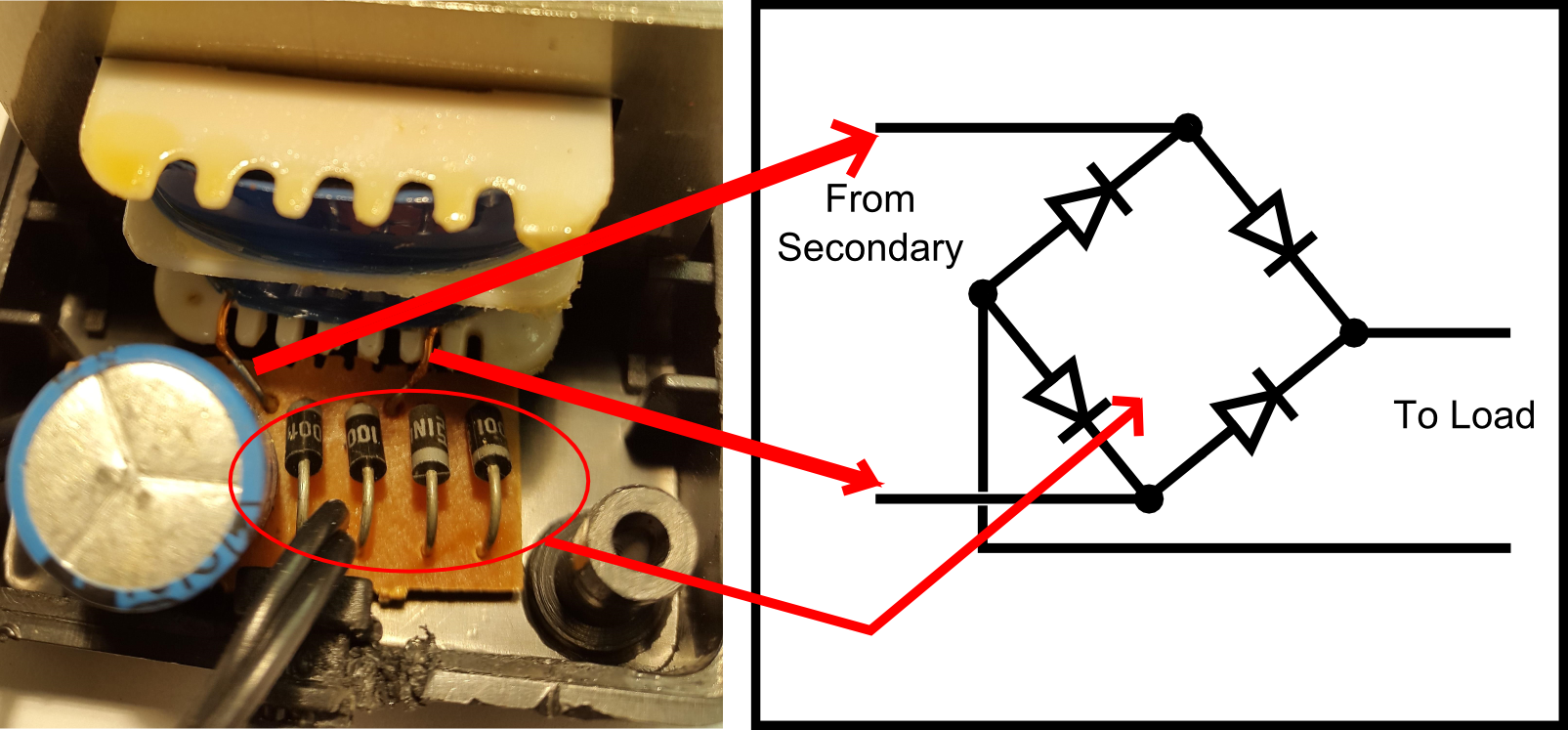Figure 8. Full Bridge Rectifier Circuit and Schematic

The bridge rectifier in this wall adapter is made of four individual diodes (part number 1N4001), but sometimes the rectifier is a basic integrated circuit with the four diodes manufactured all in one device like in figure 9.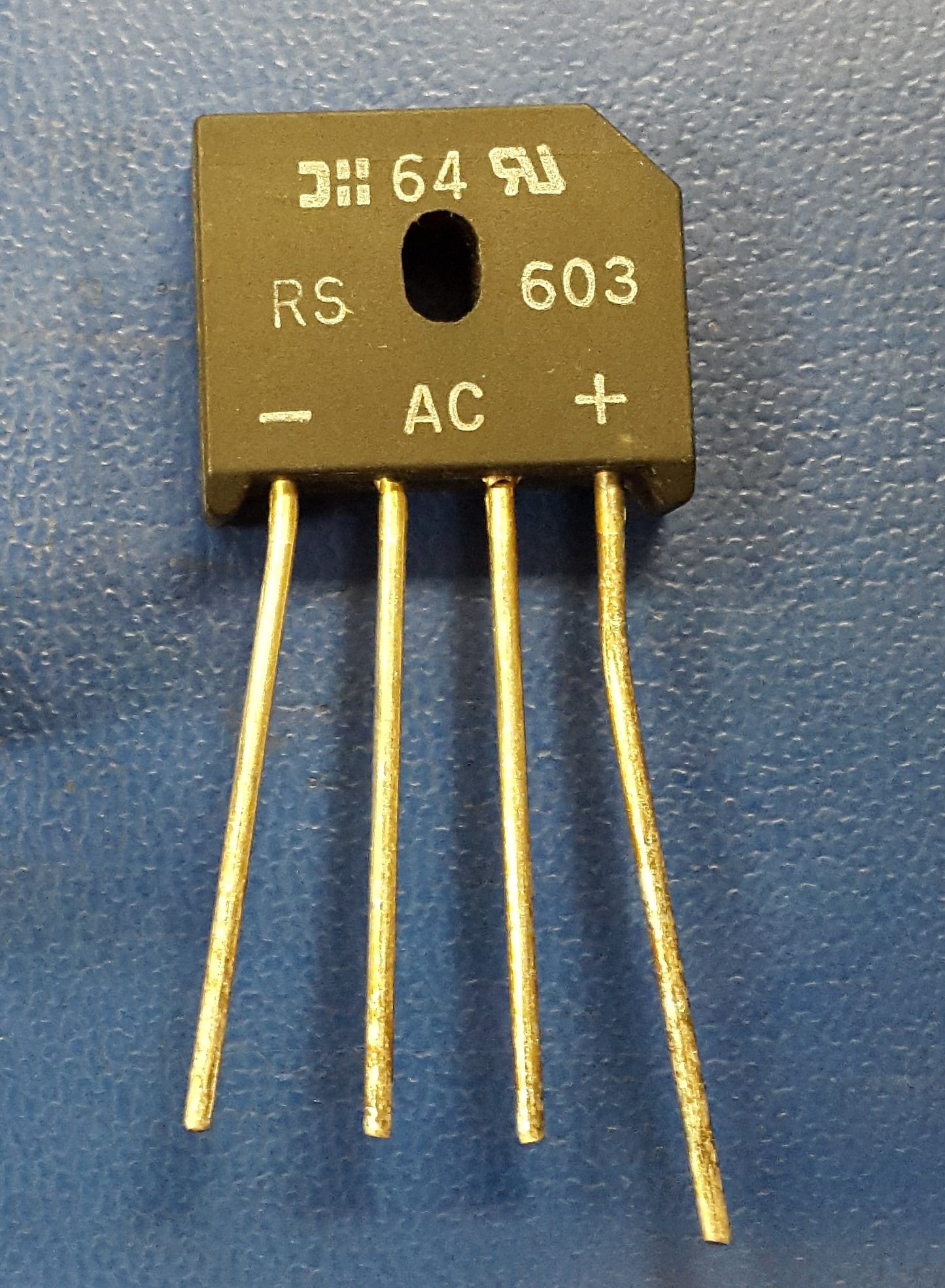Figure 9. Bridge Rectifier in an IC

The output of the rectifier is only DC in the sense that current to the load is forced in one direction. The voltage is still varying a large amount as can be seen in figure 10.  Effectively what the rectifier did was to take the negative portion of the voltage and flip it around to make it positive as shown in the figure below. The voltage still swings between 0V and the peak. Further processing must be done on the voltage to minimize the voltage swing and that is what the next stage does.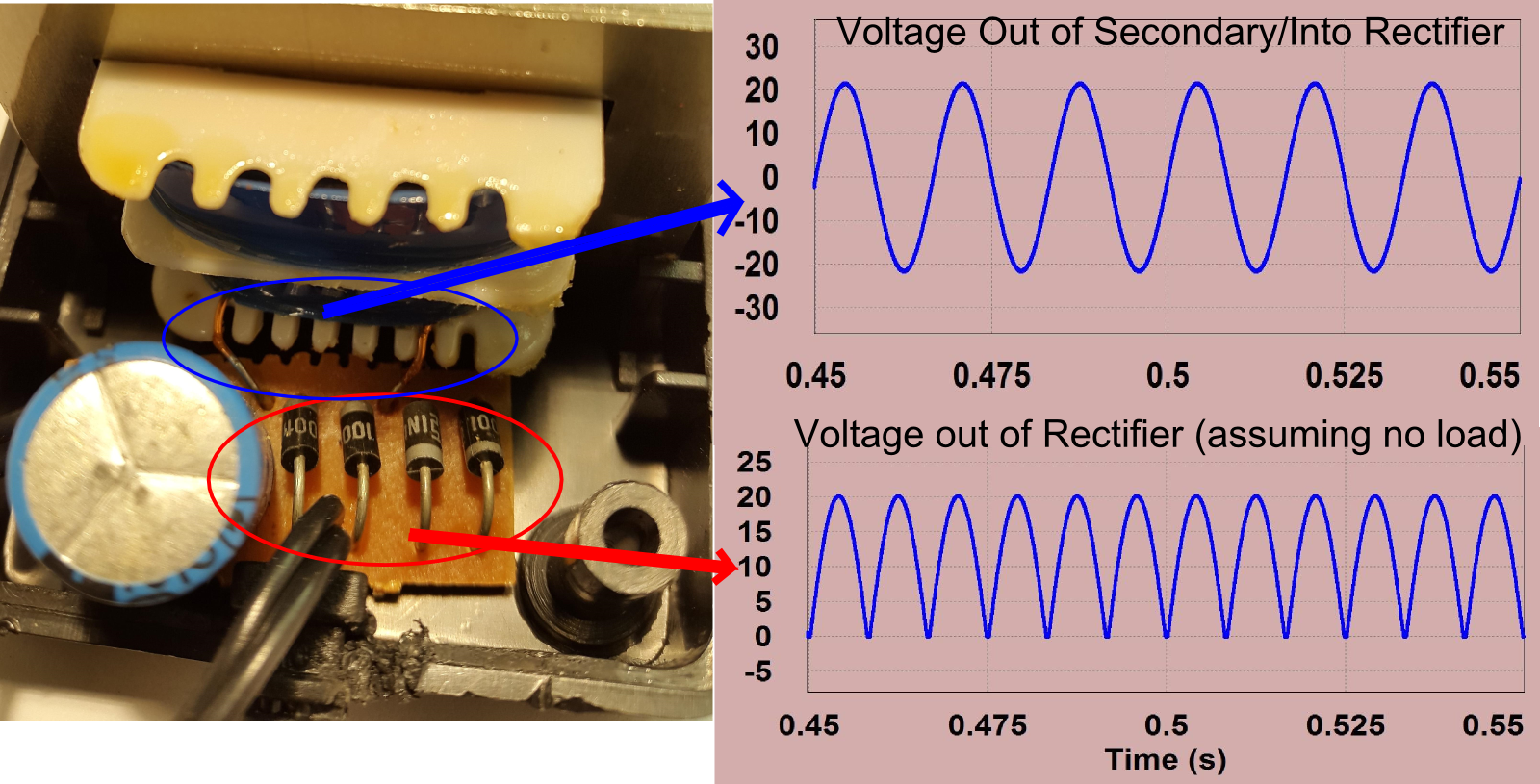Figure 10. Rectifier Circuit Showing Input and Output Voltages.png

### The Capacitor

The next problem to solve is how to take that varying voltage and smooth it out so that the load receives a more or less constant voltage. The main component in this fight against this ripple is the capacitor. The capacitor is the tall blue cylindrical component in figure 11 below: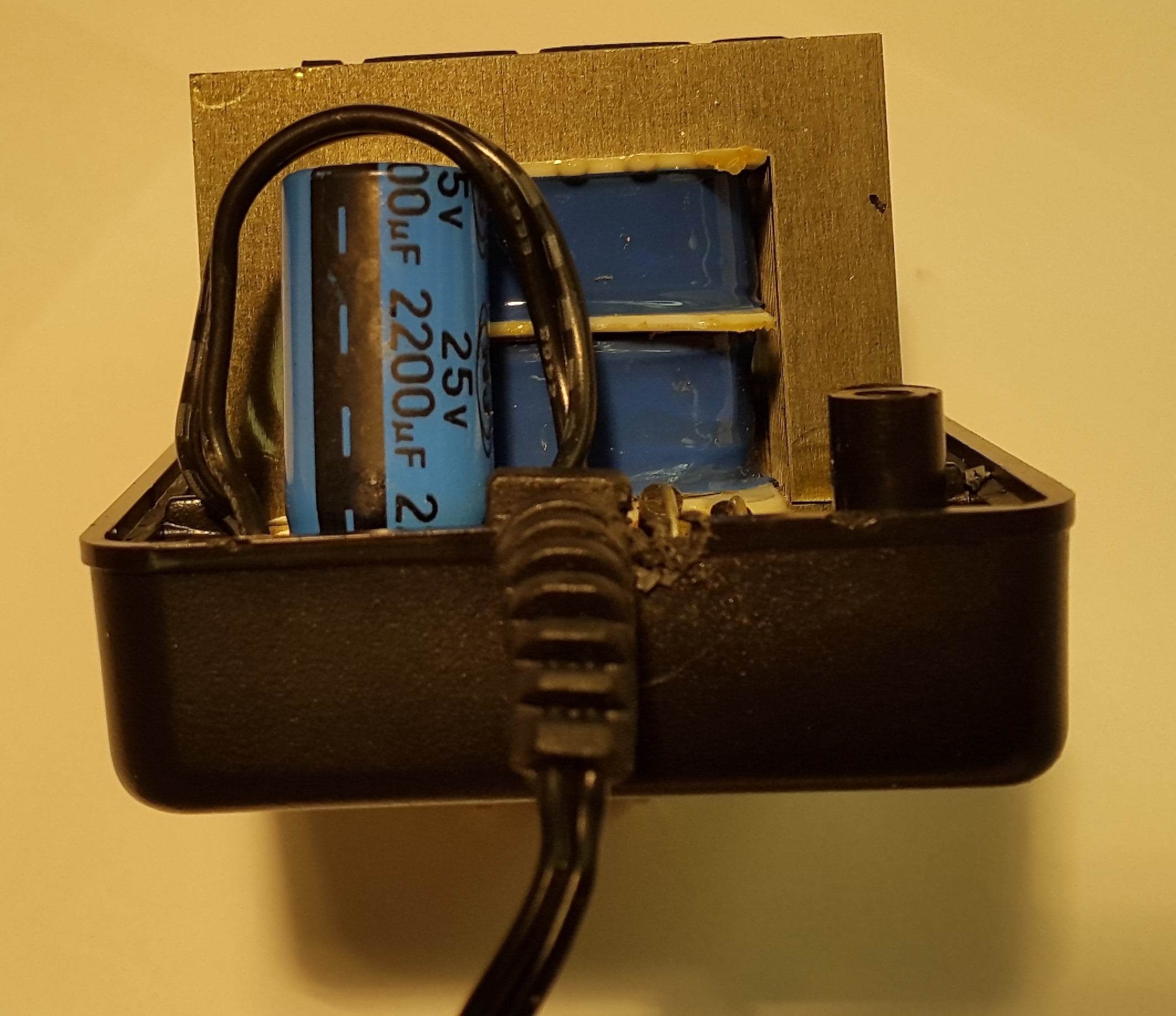Figure 11. Capacitor in AC Adaptor

The capacitor in this wall adapter is a 2200 uF electrolytic capacitor. Electrolytic capacitors are typically used because it is possible to have a relatively high capacitance (100s or even 1000s of uF) and reasonable voltage tolerance (10’s of volts) at an affordable price. For example, a quick search on an electronic component supplier’s website shows me that a 2200 uF capacitor that can tolerate up to 50V is under $3 if it is an electrolytic capacitor and more than$250 if it is a film capacitor. The primary downside of electrolytic capacitors is that they have a much shorter life expectancy than film capacitors. In fact electrolytic capacitors are likely to be the component that fails first in any electronic system. Generally manufacturers ignore this reliability issue because using electrolytic capacitors results in such a big cost savings. However in certain circuits where reliability is very important film capacitors are considered.

The purpose of the capacitor is to attempt to keep the voltage to the load constant by absorbing extra energy from the source when the source is providing more energy than the load needs and outputting extra energy to the load when the source is providing less energy than the load needs. The result of this filtering on the voltage can be seen in figure 12.Figure 12.  AC Adaptor Output Voltage With and Without Filter Capacitor

Notice that the voltage is still not a constant voltage, there is a little bit of ripple at the output. There will always be a small amount of ripple in the voltage to the load regardless of the size of capacitor used. A larger capacitor can eliminate more ripple because it can store more energy for a given voltage. Conversely, a larger load (i.e., a smaller resistance) will lead to more ripple because more energy will be drawn from the capacitor during times of need causing it to discharge more. It is easy to calculate how much ripple you will have given a particular capacitance ($C$), load ($R_{L}$), and frequency ($f$).

$V_{ripple(p-p)} = \frac{I_{DC}}{Cf} = \frac{V_{DC}}{R_{L}Cf}$

Alternatively it is easy to calculate how much capacitance you will need to meet a certain ripple specification given a particular load. The equation looks like this:

$C = \frac{I_{DC}}{V_{ripple(p-p)}f} = \frac{V_{DC}}{V_{ripple(p-p)}R_{L}f}$

One final note about the capacitor in the circuit. The capacitor is able to keep the voltage relatively steady with a little bit of ripple, but the current into and out of the capacitor is not very steady at all. Figure 13 shows the current into and out of the capacitor (Icap) as it charges and discharges.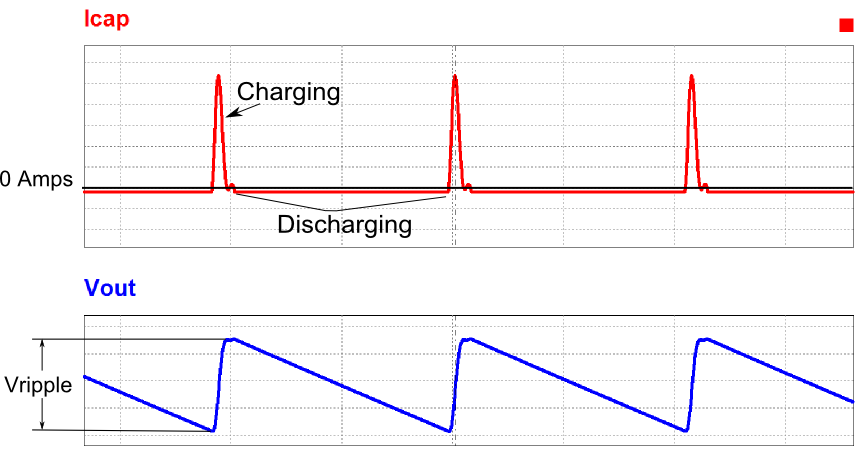Figure 13.  Charging and Discharging Capacitor Current

Notice how the charging cycle is a very short burst of high current from the source while the discharging cycle is a slow and steady flow of current to the load. You can also see how the output voltage jumps up during the current burst to charge the capacitor and slowly drops during the capacitor discharge. These current bursts can lead to problems such as a low power factor and high harmonic distortion. In low power converters like this one, these problems can typically be ignored, but in higher power converters extra circuitry may need to be added to correct these problems.

### Full Circuit Recap

The preceding sections of this article show that the transformer, the rectifier and the capacitor are all that are required for a basic AC-DC converter. This final picture and schematic shows the end to end voltage processing done by the converter as it converts AC voltage into DC voltage.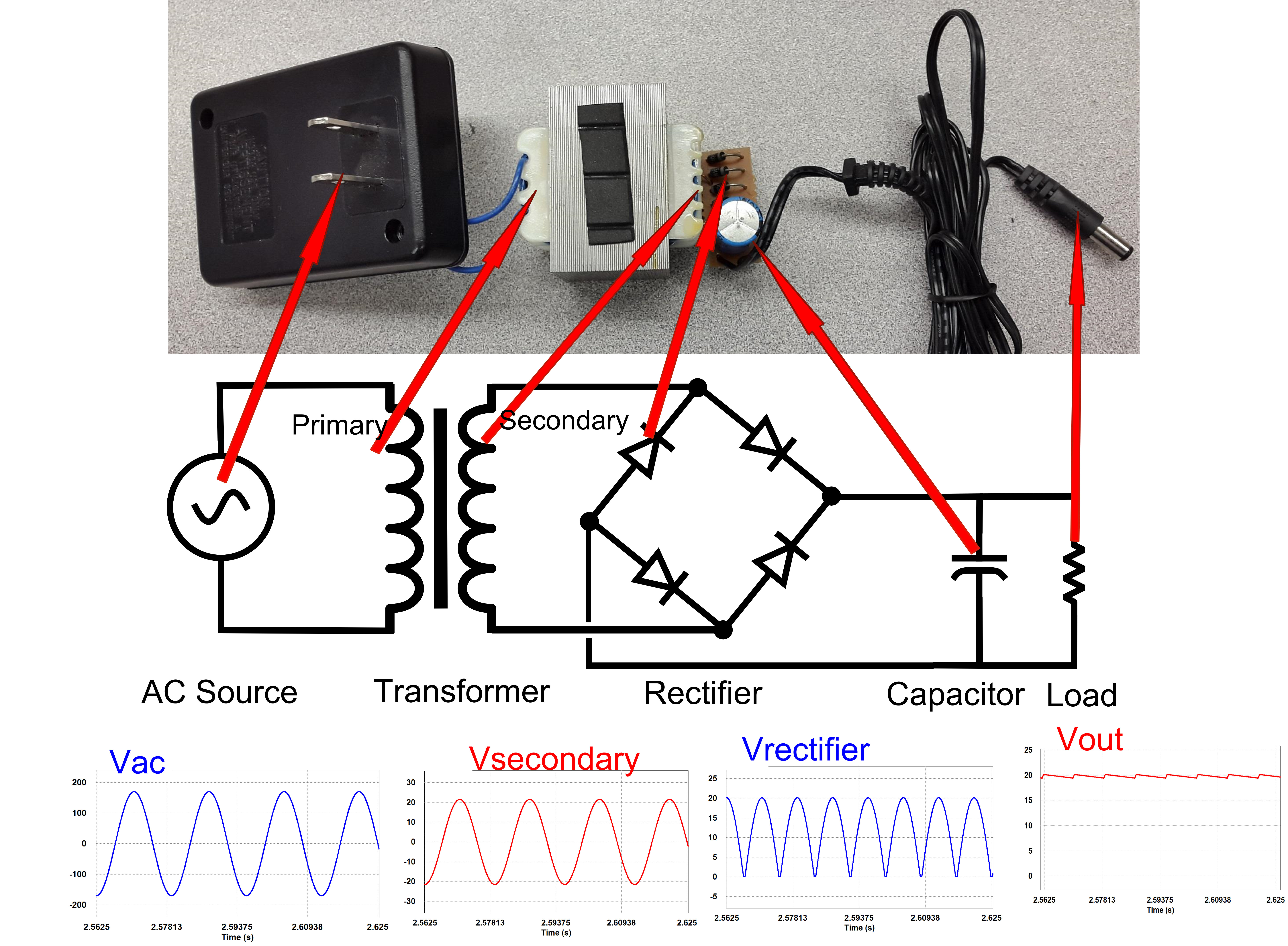Figure 14. AC Adaptor, Schematic and Voltages

From this picture it looks like we get a reasonably steady DC output voltage given a 120VRMS AC input voltage (note that the output is unregulated, so with no load, the DC voltage is actually higher than the rated 12V). For this 20 watt AC-DC converter, as long as the voltage ripple is meeting your specifications, there is not much more that you need to worry about. However, as mentioned earlier, there can be problems at higher powers due to the large in-rush current to the capacitor as it is recharged.  These problems will be analyzed in part 2 of the rectifier investigation.•jlachance December 31, 2015

Nice article, Dave!  Looking forward to part 2!

Like.
•MWUsman January 02, 2016

Quite resourceful! We shall but follow to the end.

Like.
• J
jkras4540 January 15, 2016

Good presentation, keep the formulas coming.

Like.
• L
LEhenson May 20, 2016

Now add an LM317 (or LM 350), small electrolytic cap and variable resistor, = variable power supply!

Like.
• F
fatima majdee June 04, 2016

But how can i use 2 input voltage sources with one switch, and one transformer?

Like.
•etech7 June 07, 2016
You can use center tapped transformer. from bridge rectifier you will get full voltage of secondary and between rectifier and center tap you will get half voltage that of secondary
Like.
•Louis Wilen June 19, 2016

Very good article with excellent illustrations.

As you mentioned, this particular power supply uses a bridge rectifier.  I’ve seen some that use just a single diode for rectification, which of course results in half-wave DC output.  It’s amazing what a manufacture will (not) do to save just a few cents.

Like.
• C
charles2014 August 20, 2016

So powerfully, its nice one

Like.
•Harshvir Singh July 30, 2017

Great article! but I’d really appreciate it if you could elaborate on WHY the capacitor current is so haywire

Like.